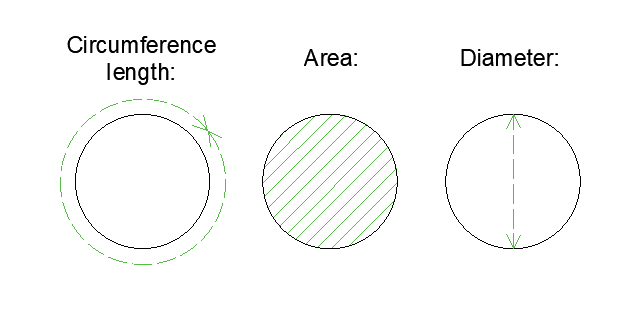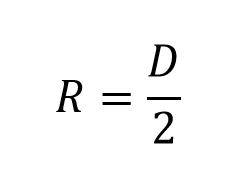# Calculator circle radio, formulas, examples and definitions

To calculate the radius of a circle, three different formulas can be used, depending on the Area, the diameter or the length of the circumference.

But do not worry, with this calculator you can know the radio automatically, if you want more details we also have the formulas and some examples.## Formulas to calculate the radius of a circle:

1. Formula to calculate the radius from the area.  The following formula is used to calculate the radius, when you have the area of ​​the circle:Where:

• A:  Area.
• π: Constant Number equivalent to 3.1415

2. Formula for calculating the radius when you have the diameter.  When we have the diameter we can calculate the radius by means of the following conversion formula:Where:

• D:  Diameter.

3. Formula to perform the calculation of the radius from the length of the circumference. If for some reason you only have the length of the circumference, with this data and the following formula you can find the radius:Where:

• LC: Length of the circumference.
• π: Constant Number equivalent to 3.1415

## Steps to calculate the radius of a circle:

The above formulas can be used to calculate the radius easily and quickly, the formula you use depends on the data you have (Diameter, area or circumference length):

### – How is the radius of a circle calculated when you have the area ?:

Step 1.  Once you have the value of the area you should divide it between the constant pi (π), giving you as a result a number. Example: you have an area of ​​34, divide between (π) and the result will be: 10,822

Step 2.  Then you should take the number from step 1 and take the square root as follows: √10,822 = 3,289.

### – How to calculate the radius of a circle if you have the diameter ?:

Step 1. This is the simplest formula, because it only requires one step, because it is as simple as dividing in diameter between 2. Example: If you have a diameter of 23, you only have to divide 23 between two and the result will be the radius : 23/2 = 11.5

### – How is the radius of a circle calculated if I have the length of the circumference ?:

Step 1.  Multiply first 2xπ, the result will always be: 6,283

Step 2. In this case it is essential to have the length of the circumference. Because you must divide the length of the circumference between the value of step 1. Example: You have a length of the circumference of 42, this value is divided as follows: 42 / 6,283 = 6,684

[kkstarratings]